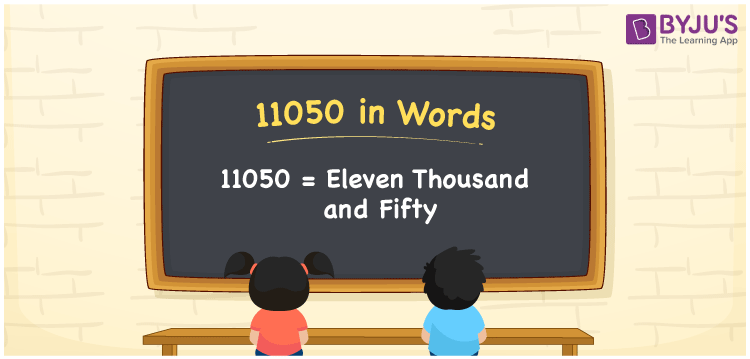# 11050 in Words

11050 in words is eleven thousand and fifty. Using the place values of each digit of the number, we can easily write the number name 11050. Also, the number 11050 is a cardinal number. For example, there are 11050 books in a library. Now, let us learn the method of writing the number 11050 in words in this article.

 11050 in Words: Eleven Thousand and Fifty. Eleven Thousand and Fifty in Numerical Form: 11050.

## 11050 in English Words## How to Write 11050 in Words?

The place value table for the 5 digit number 11050 is as follows:

 Ten-thousands Thousands Hundreds Tens Ones 1 1 0 5 0

The expanded form of 11050 is as follows:

= 1 × Ten thousand + 1 × Thousand + 0 × Hundred + 5 × Ten + 0 × One

= 1 × 10000 + 1 × 1000 + 0 × 100 + 5 × 10 + 0 × 1

= 10000 + 1000 + 50

= 11050

= Eleven thousand and fifty

Hence, 11050 in words is eleven thousand and fifty.

11050 in words – Eleven thousand and fifty

Is 11050 an odd number? – No

Is 11050 an even number? – Yes

Is 11050 a perfect square number? – No

Is 11050 a perfect cube number? – No

Is 11050 a prime number? – No

Is 11050 a composite number? – Yes

## Frequently Asked Questions on 11050 in Words

Q1

### How to write 11050 in words?

11050 in words is eleven thousand and fifty.

Q2

### Simplify 11000 + 50, and express it in words.

Simplifying 11000 + 50, we get 11050. Hence, 11050 in words is eleven thousand and fifty.

Q3

### Is 11050 an even number?

Yes, 11050 is an even number.